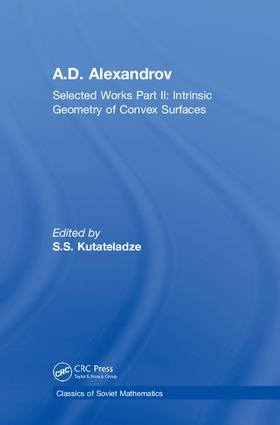# A.D. Alexandrov

## Selected Works Part II: Intrinsic Geometry of Convex Surfaces, 1st Edition

Chapman and Hall/CRC

444 pages | 100 B/W Illus.

##### Purchasing Options:\$ = USD
Hardback: 9780415298025
pub: 2005-07-25
SAVE ~\$43.00
\$215.00
\$172.00
x
eBook (VitalSource) : 9780429212437
pub: 2005-07-25
from \$28.98

FREE Standard Shipping!

### Description

A.D. Alexandrov is considered by many to be the father of intrinsic geometry, second only to Gauss in surface theory. That appraisal stems primarily from this masterpiece--now available in its entirely for the first time since its 1948 publication in Russian.

Alexandrov's treatise  begins with an outline of the basic concepts, definitions, and results relevant to intrinsic geometry. It reviews the general theory, then presents the requisite general theorems on rectifiable curves and curves of minimum length. Proof of some of the general properties of the intrinsic metric of convex surfaces follows. The study then splits into two almost independent lines: further exploration of the intrinsic geometry of convex surfaces and proof of the existence of a surface with a given metric. The final chapter reviews the generalization of the whole theory to convex surfaces in the Lobachevskii space and in the spherical space, concluding with an outline of the theory of nonconvex surfaces.

Alexandrov's work was both original and extremely influential. This book gave rise to studying surfaces "in the large," rejecting the limitations of smoothness, and reviving the style of Euclid. Progress in geometry in recent decades correlates with the resurrection of the synthetic methods of geometry and brings the ideas of Alexandrov once again into focus. This text is a classic that remains unsurpassed in its clarity and scope.

### Reviews

“This classic is quite readable and opens a deeper understanding of this field also through self-study without any special prerequisites …”

— H. Rindler, Wien, in Monatshefte für Mathematik, Vol. 149, No. 4, 2006

### Table of Contents

BASIC CONCEPTS AND RESULTS

The General Concept of Intrinsic Geometry and Its Problems

Gaussian Intrinsic Geometry

A Polyhedral Metric

Development

Passage from Polyhedra to Arbitrary Surfaces

A Manifold with Intrinsic Metric

Basic Concepts of Intrinsic Geometry

Curvature

Characteristic Properties of the Intrinsic Metric of a Convex Surface

Some Special Features of Intrinsic Geometry

Theorems of Intrinsic Geometry of Convex Surfaces

General Propositions about the Intrinsic Metric

General Theorems on Rectifiable Curves

General Theorems on Shortest Arcs

Nonoverlapping Condition for Shortest Arcs

A Convex Neighborhood

General Properties of Convex Domains

Triangulation

CHARACTERISTIC PROPERTIES OF THE INTRINSIC METRIC

Convergence of Metrics of Convergent Convex Surfaces

Convexity Condition for a Polyhedral Metric

Convexity Condition for the Metric of a Convex Surface

Consequences of the Convexity Condition

ANGLE

General Theorems on Addition of Angles

Theorems on Addition of Angles on Convex Surfaces

The Angle of a Sector Bounded by Shortest Arcs

On Convergence of Angles

The Tangent Cone

The Spatial Meaning of the Angle Between Shortest Arcs

CURVATURE

Intrinsic Curvature

Area of the Spherical Image

Generalization of the Gauss Theorem

Curvature of Borel Sets

Set of Directions in Which It Is Impossible to Draw a Shortest Arc

Curvature as a Measure of Non-Euclidicity of Space

EXISTENCE OF A CONVEX POLYHEDRON WITH A GIVEN METRIC

On Determination of a Metric from a Development

The Idea of the Proof of the Realization Theorem

Small Deformations of a Polyhedron

Deformation of a Convex Polyhedral Angle

Rigidity Theorem

Realizability of the Metrics That Are Close to the Realized Metrics

Smooth Passage from a Given Metric to a Realizable Metric

Proof of the Realization Theorem

EXISTENCE OF A CLOSED CONVEX SURFACE WITH A GIVEN METRIC

The Result and the Method of Proof

The Main Lemma on Convex Triangles

Consequences of the Main Lemma on Convex Triangles

The Complete Angle at a Point

Curvature and Two Related Estimates

Approximation of a Metric of Positive Curvature

Realization of a Metric of Positive Curvature Given on the Sphere

OTHER EXISTENCE THEOREMS

Glueing Theorem

Application of the Glueing Theorem to the Realization Theorems

Realizability of a Complete Metric of Positive Curvature

Manifolds on Which a Metric of Positive Curvature Can Be Given

Uniqueness of a Convex Surface

Various Definitions of a Metric of Positive Curvature

CURVES ON CONVEX SURFACES

The Direction of a Curve

The Swerve of a Curve

General Glueing Theorem

Convex Domains

Quasigeodesics

A Circle

AREA

The Intrinsic Definition of Area

The Extrinsic-Geometric Meaning of Area

Extremal Properties of Pyramids and Cones

THE ROLE OF SPECIFIC CURVATURE

Intrinsic Geometry of a Surface

Intrinsic Geometry of a Surface of Bounded Specific Curvature

Shape of a Convex Surface in Dependence on Its Curvature

GENERALIZATION

Convex Surfaces in Spaces of Constant Curvature

Realization Theorems in Spaces of Constant Curvature

Surfaces of Indefinite Curvature

BASICS OF CONVEX BODIES

Convex Domains and Curves

Convex Bodies. A Supporting Plane

A Convex Cone

Topological Types of Convex Bodies

A Convex Polyhedron and the Convex Hull

On Convergence of Convex Surfaces

### Subject Categories

##### BISAC Subject Codes/Headings:
MAT000000
MATHEMATICS / General
MAT012000
MATHEMATICS / Geometry / General